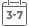# Markov Processes and Applications: Algorithms, Networks, Genome and Finance

• Publish Date: 2009-01-07
• Binding: Hardcover
• Author: Etienne Pardoux
• Sale
• \$50.25
• Regular price \$54.60This well-written book provides a clear and accessible treatment of the theory of discrete and continuous-time Markov chains, with an emphasis towards applications. The mathematical treatment is precise and rigorous without superfluous details, and the results are immediately illustrated in illuminating examples. This book will be extremely useful to anybody teaching a course on Markov processes.
Jean-Franois Le Gall, Professor at Universit de Paris-Orsay, France
.

Markov processes is the class of stochastic processes whose past and future are conditionally independent, given their present state. They constitute important models in many applied fields.

After an introduction to the Monte Carlo method, this book describes discrete time Markov chains, the Poisson process and continuous time Markov chains. It also presents numerous applications including Markov Chain Monte Carlo, Simulated Annealing, Hidden Markov Models, Annotation and Alignment of Genomic sequences, Control and Filtering, Phylogenetic tree reconstruction and Queuing networks. The last chapter is an introduction to stochastic calculus and mathematical finance.

Features include:

• The Monte Carlo method, discrete time Markov chains, the Poisson process and continuous time jump Markov processes.
• An introduction to diffusion processes, mathematical finance and stochastic calculus.
• Applications of Markov processes to various fields, ranging from mathematical biology, to financial engineering and computer science.
• Numerous exercises and problems with solutions to most of them

MORE FROM THIS COLLECTION